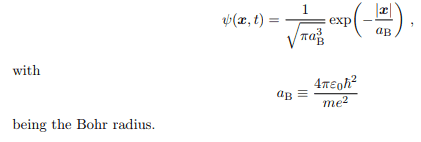# Expectation value <p> of the ground state of hydrogen

Warda Anis

## Homework Statement

How should I calculate the expectation value of momentum of an electron in the ground state in hydrogen atom.

## Homework Equations## The Attempt at a Solution

I am trying to apply the p operator i.e. ##-ihd/dx## over ##\psi##. and integrating it from 0 to infinity. The answer I am getting is ##ih/{2(pi)a^3}##

## Answers and Replies

Homework Helper
Gold Member
2021 Award
You are actually trying to calculate the expectation value of px. To do that, you must express the ground state wavefunction explicitly as a function of ##x## so that you can take the derivative. You have to do a 3d integral.

•jedishrfu
Warda Anis
So I did the following to integrate it in 3d:$$\iiint_V \Psi^* (-i\hbar) \frac {d\Psi} {dr} r^2 sin\theta dr d\theta d\phi$$

The final answer i am getting is ##\frac {i\hbar}{a_b}## which does not look right because it is imaginary and momentum operator is hermitean. I can't figure out what mistake I am doing

•